## New Maths Curriculum: Year 2 Multiplication and Division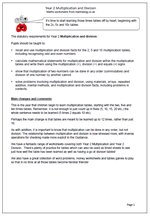Year 2 Multiplication and Division

It’s time to start learning those times tables off by heart; beginning with the 2x, 5x and 10x tables.
The statutory requirements for Year 2 Multiplication and division :

Pupils should be taught to:

• recall and use multiplication and division facts for the 2, 5 and 10 multiplication tables, including recognising odd and even numbers
• calculate mathematical statements for multiplication and division within the multiplication tables and write them using the multiplication (×), division (÷) and equals (=) signs
• show that multiplication of two numbers can be done in any order (commutative) and division of one number by another cannot
• solve problems involving multiplication and division, using materials, arrays, repeated addition, mental methods, and multiplication and division facts, including problems in contexts.

This is the year that children begin to learn multiplication tables, starting with the two, five and ten times tables. Remember, it is not enough to just count up in fives (5, 10, 15, 20 etc.) the whole sentence needs to be learned (5 times 2 equals 10 etc).
Perhaps the main change is that tables are meant to be learned up to 12 times, rather than just 10.

As with addition, it is important to know that multiplication can be done in any order, but not division. The relationship between multiplication and division is now stressed more, with inverse operations for checking made more explicit in the Guidance.

Year 2 multiplication and division statutory requirements

## New Maths Curriculum: Year 1 Multiplication and Division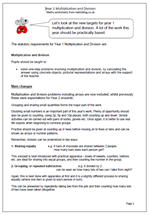Let’s look at the new targets for year 1 multiplication and division. A lot of the work this year should be practically based.

The statutory requirements for Year 1 Multiplication and Division are:

Multiplication and division

Pupils should be taught to:

•    solve one-step problems involving multiplication and division, by calculating the answer using concrete objects, pictorial representations and arrays with the support of the teacher.

Main changes

Multiplication and division problems including arrays are now included, whilst previously these were expectations for Year 2 onwards.

Grouping and sharing small quantities forms the major part of the work.

Doubling small numbers is an important part of this year’s work. Plenty of opportunity should also be given to counting, using 2p, 5p and 10p pieces, both counting up and down. Similar activities can be carried out with pairs of socks, gloves etc. Once again, it is better to use real life objects when beginning to combine groups.

Practice should be given at counting up in twos before moving on to fives or tens and can be shown as arrays or number patterns.
Remember, division can be understood in two ways:

1. Sharing equally               e.g. 6 bars of chocolate are shared between 2 people.
How many bars does each person get?

This concept is best introduced with practical apparatus – bowls of sweets, counters, buttons etc. are ideal for sharing into equal groups, and then counting the number in the group.

2. Grouping, or repeated subtraction                     e.g. 8 divided by 2
can be seen as how many lots of two can I take from eight?

Again, this is best done with apparatus at first and it is a slightly different process to sharing equally (where one item is given to each person in turn).

This can be answered by repeatedly taking two from the pile and then counting how many lots of two have been taken altogether.

Multiplication and division in Year 1

## Mental division practice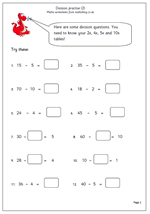being able to divide mentally is essential and is made much easier by knowing times tables.

Here is a follow up page to one published earlier, giving more practice with simple division. If children have a good knowledge of the 2x, 4x, 5x and 10x tables they should find these quite straightforward. The only potentially tricky ones are where the missing number is in the middle of the number sentence

eg 24 divided by ? = 6

This requires a good understanding of what the number sentence means, but all that is required to answer is a knowledge of ‘what multiplied by 6 makes 24’.

This worksheet can be found in our Year 3 Knowing Number Facts section.

Y3 division practice 2

## Year 5 Knowing multiplication facts to help with division.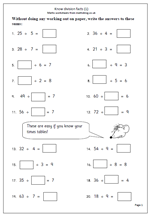There is no doubt that most children find division harder than multiplication, yet there is little real reason for this to be so. Most division questions can be turned on their head and knowledge of times tables used to work them out. For example:

30 ÷ 5 =??  can be thought as what number times 5 makes 30?

?? ÷ 6 = 7 can be thought of as 6 times 7.

60 ÷ ?? = 10 can be thought of as what number times 10 makes 60?

The key to success with division is to have a really good knowledge of times tables.

This worksheet looks at these types of division question, all with easy numbers and no remainders and is a good assessment sheet to see if division is understood and tables known. if times tables are not known sufficiently well children will spend more time than necessary trying to work these out.

This can be found in our Year 5 Knowing Number Facts category.

Know division facts (1)

## Resource of the Week: short division of decimals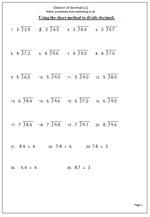This week I would like to look at a page of practice on using the short method of division of decimals. The numbers being divided are just units and tenths which helps with getting the method correct.

There are arguments for and against putting the decimal point in before you start, or leaving it until you have reached that point in the question. it does not matter as long as it is inserted correctly.

One of the best ways to be fluent with this method is to talk it through out loud. If we look at question 2 which is 4.5 divided by 3, the verbal stages are:

a. How many 3s in 4?

b. 1 times 3 is 3 so there is 1 with a remainder of 1.

c. Place the 1 on the answer line, immediately above the 3.

d. Place the decimal point just above the answer line so it can be clearly seen.

e. The remainder 1 is placed just in front of the 5 (usually written smaller).

f. How many 3s in 15?

g. 3 x 5 is 15 so the answer is 5.

h. Place the 5 on the answer line, immediately above the 5 (tenths).

Division of decimals (1)

## Division: 3-digit numbers divided by 8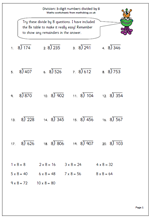Here is another page for practising the short division method, this time just using the eight times table. The key to this method is, of course, a good knowledge of the 8 times table, as without this the solution can take an awfully long time and lots of errors may occur. The table has been written out as a helpful starting point, so that the method can be concentrated on.

For example: 8)659

‘How many eights in 65?’

‘8 times 8 is 64, 9 times 8 is 72 which is too much.’

‘8 goes into 65, 8 times with 1 left over.’

Write the 8 in the answer above the 5.

Write the remainder 1 beside the 9 units, making 19.

‘How many eights in 19?’

‘2 times 8 is 16.’

‘8 goes into 19, 2 times with 3 left over.’

Write the 2 in the answer in the units and write rem 3 next to it.

Division: 3 digits by 8

## Year 5 division problems: calculating mentally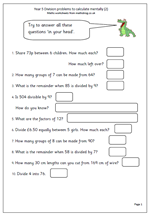Here is the second page which looks at Year 5 division problems that can be answered by using mental skills. The questions cover a range of concepts involving division, including:

remainders

divisibility rule for 9

and factors.

Watch out for errors that occur in questions such as number 9, where the number of whole lengths of wire is calculated and the remainder is irrelevant.

This page, and other similar worksheets can be found in the Year 5 calculating category.

Division problems (2)

## Resource of the week: division as sharing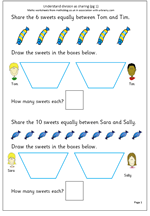Our resource of the week this week looks at early understanding of division. Division is usually the hardest of the four rules for children to learn, but in the early stages it is quite straightforward. Children often come across division for the first time when sharing, usually between two. The key concept,of course, is that the sharing is done equally. So, for example, 6 sweets shared between 2 children implies that the sharing is equal and they both receive the same number.

By far the best way to practice sharing is to use practical apparatus or use real life situations: e.g. share the strawberries equally between two, share or deal the cards equally etc. Usually this is done on a ‘one for you and one for me’ type process until there are none left. This maths worksheet replicates a practical situation, with the ultimate aim that children begin to remember the answers, which, of course are the inverse of multiplication.

Be careful when sharing that it is not always done into two, as some children begin to think that to share something it can always be divided by 2 and no other number.

Division as sharing (pg 1)

## Dividing by 3 table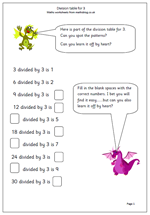In recent years there has been a call for children to not only learn the times tables but also the equivalent division tables. Of course this is the inverse of the 3x table and for children who know this there should not be much of a problem learning this. There are advantages to be had as it can lead to quicker mental arithmetic when carrying out written long and short division. So, here have have a quick look at learning facts connected to dividing by three and an exercise putting this knowledge into practice.

Divide by 3 table

## Divide 3-digit numbers by 6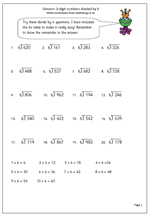This is a short division page which concentrates just on dividing 3-digit numbers by 6. Short division, as its name suggests is a shortened version of the long division method where subtractions and remainders are done mentally.

The key to answering these is to have a set speech for the process.

For example: 6)457

‘How many sixes in 45?’

‘7 times 6 is 42.’

‘6 goes into 45, 7 times with 3 left over.’

Write the 7 in the answer above the 5.

Write the remainder three beside the 7 units, making 37.

‘How many sixes in 37?’

‘6 times 6 is 36.’

‘6 goes into 37, 6 times with 1 left over.’

Write the 6 in the answer in the units and write rem 1 next to it.

Division: 3-digit numbers by 6